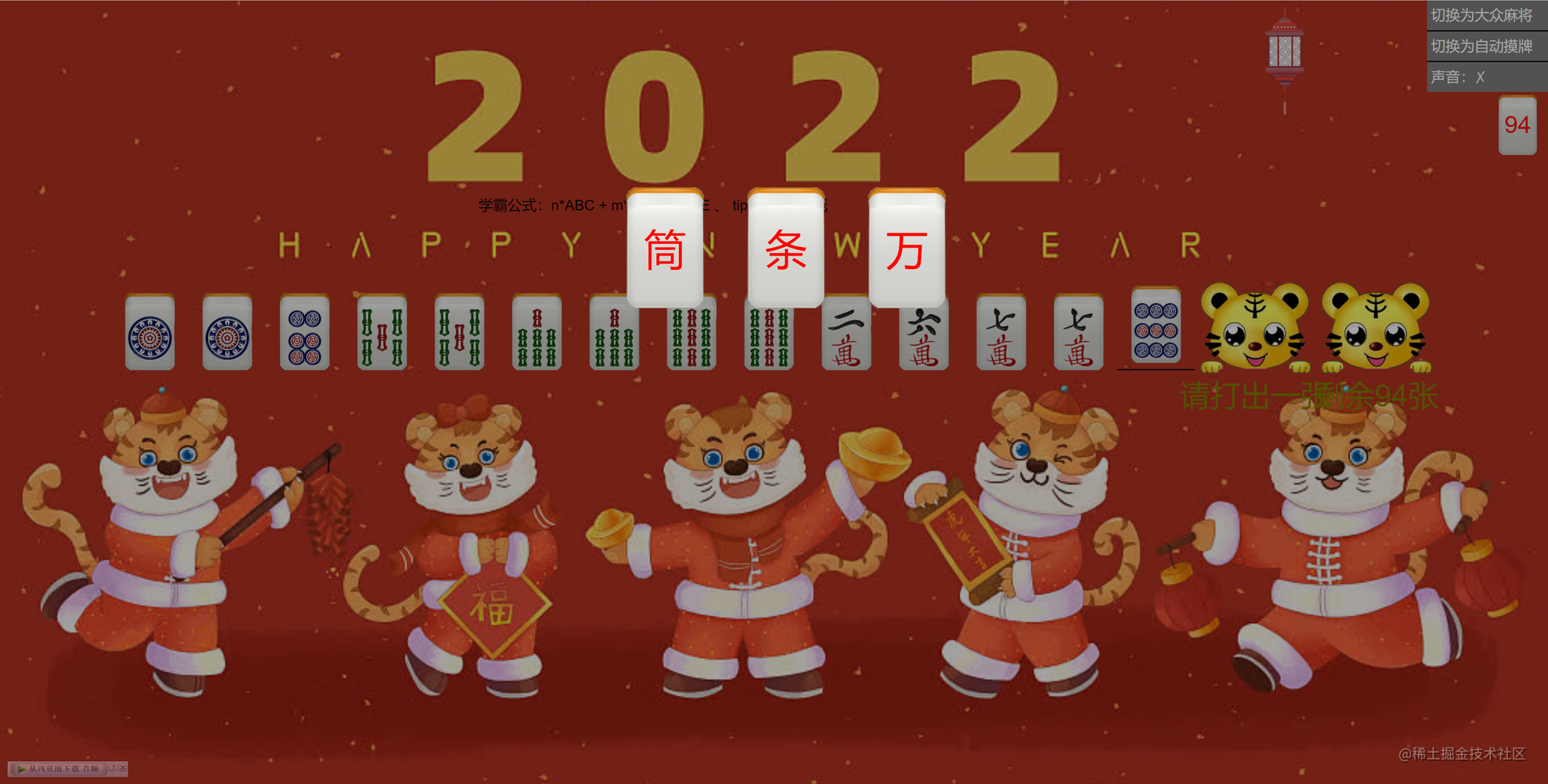# 都て0てて年了，还有人不会打麻将？gitee地址：gitee.com/yan_yin/mah…

## 游戏规则

`ABC:顺子 、DDD:三个相同 、EE:对子 | n>=0 、m>=0`## 菜单及全局配置

### 模式切换

``````<div class="container">
<da-zhong v-if="!model" />
<si-chuan v-else />
</div>

### 自动摸牌

``````const delMj =  (index)=> {
if([1,4,7,10,13].includes(data.handMj.length)){
}else{
data.handMj.splice(index,1)
//摸牌的时候不排序  打出去的时候在排
handMjSort()
}
}

``````  const handMjSort = ()=> {
setTimeout(()=> {
data.handMj.sort((a,b )=>a-b)
//自动摸牌
if(Number(localStorage.getItem('model2'))==1)getNewMj()
},1000)
}
const getNewMj = ()=> {
//摸牌后排序
data.handMj.push(...data.remainMj.splice(0,1))
checkMj()
//手牌麻将重复数最多的麻将
let max = findNum(data.handMj)
//是否有杠
if(max==4){
//有杠
data.isGang = true
data.gangId = max
}else{
data.isGang = false
}

}

### 声音

``````<audio hidden="true" ref="audio" loop controls>
<source src="./assets/woop.mp3" type="audio/mpeg" />
</audio>

``````const audio = ref();
audio.value.volume = 0.1
audio.value.play()
audio.value.pause()

## 思路

### 麻将ID

``````for (let i = 1; i <28;i++) {
for (let j = 0; j <4;j++) {
//将  筒 条 万不同花色的隔开
if(i<=9){
mjArr.push(i)
}else if(i<=18){
mjArr.push(i+1)
}else if(i<=27){
mjArr.push(i+2)
}
}
}
mjArr = mjArr.sort(()=>0.5 - Math.random())

### 流程

``````const data = reactive({
mjArr,
selectMj:[],   //获取随机的13个
remainMj:[], //除去13个剩余的无序麻将
handMj:[],//手牌，除去杠的牌
footMj:[],//碰或者杠的牌，
isGang:false, //是否可以杠
gangId:'', //杠的id
isCheck:false, //是否胡牌
isNone:'none',//是否展示胡了图片
})
onMounted(()=> {
data.selectMj = mjArr.slice(0, 13)
data.remainMj = mjArr.slice(13)
data.handMj = [...data.selectMj]
data.handMj.sort((a,b )=>a-b)
getNewMj()
})

``````const getNewMj = ()=> {
data.handMj.push(...data.remainMj.splice(0,1))
checkMj()
//手牌麻将重复数最多的麻将
let max = findNum(data.handMj)
//是否有杠
if(max==4){
//有杠
data.isGang = true
data.gangId = max
}else{
data.isGang = false
}
}

``````//打出一张
const delMj =  (index)=> {
if([1,4,7,10,13].includes(data.handMj.length)){
}else{
data.handMj.splice(index,1)
//摸牌的时候不排序  打出去的时候在排a
handMjSort()
}
}
//判断是否胡牌
const checkMj = ()=> {
let arr  = [...data.handMj].sort((a,b )=>a-b)
data.isCheck = isHu(arr)
}

### 川麻

``````//判断是否胡牌（四川模式）
const checkMj = ()=> {
if(checkPig()){
let arr  = [...data.handMj].sort((a,b )=>a-b)
data.isCheck = isHu(arr)
}
}## 胡

`1.先判断是否7对`
`2.去除一个对牌，将剩余的牌进行ABC/DDD检测，成功后将ABC/DDD删除再进行检测`
`3.还原牌后去除下一个不同的对牌在进行ABC/DDD`